## 题目描述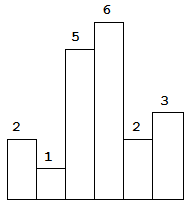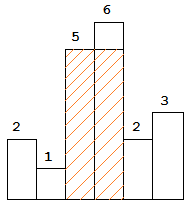## 解法

JAVA

    public int largestRectangleArea(int[] heights) {
int len = heights.length;
if (len == 0) {
return 0;
}
int ans = 0;
int[] newHeights = new int[heights.length + 2];
System.arraycopy(heights, 0, newHeights, 1, heights.length);
Deque<Integer> stack = new ArrayDeque<>();
for (int i = 0; i < newHeights.length; i++) {
while (!stack.isEmpty() && newHeights[stack.peek()]>newHeights[i]) {
int h = newHeights[stack.pop()];
int left = stack.peek() + 1;
int right = i - 1;
ans = Math.max(ans, (right - left + 1) * h);
}
stack.push(i);
}
return ans;
}


## 解题思路

### 暴力

• left指针往左寻找，直到找到大于等于当前元素高度的最左边元素的下标；
• right一个指针往右寻找，直到找到大于等于当前元素高度的最右边元素的下标；
假设当前元素的高度这个矩形的长，则两个指针的距离就是矩形的宽。
int height=heights[i];
//实际的宽度需要加一，如left=5,right=6,之间的距离实际是2
int width = right - left + 1;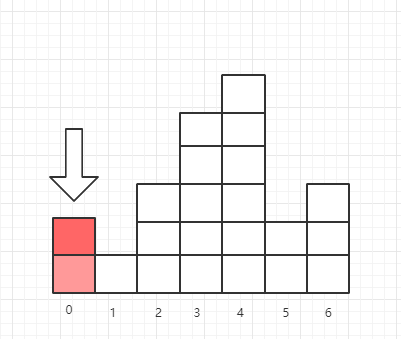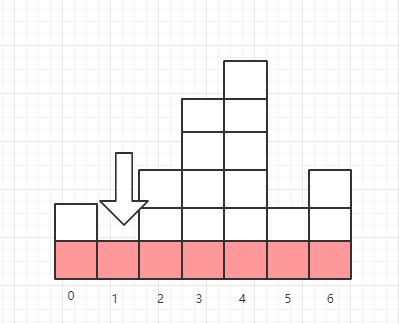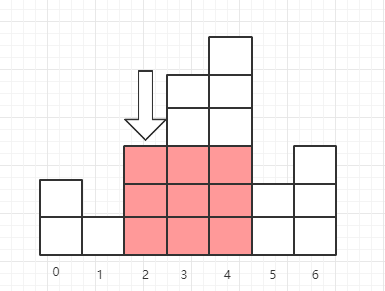##### 代码实现

 int len = heights.length;
//判断，由于题目并没有没有说明heights的长度范围，避免出现NPE
if (len == 0) {
return 0;
}
int ans= 0;
for (int i = 0; i < len; i++) {
//往左寻找
int left = i;
int height = heights[i];
while (left > 0 && heights[left - 1] >= curHeight) {
left--;
}
//往右寻找
int right = i;
//判断是否越界或者找到比他小的元素
while ((right < (len - 1) )&& heights[right + 1] >= height) {
right++;
}
int width = right - left + 1;
ans= Math.max(ans, width * height);
}
return ans;
}


### 单调栈• 当i=0，由于不知道接下来元素的情况，我们暂时记录下，temp为0；
• 当i=1时，当前元素为1，由于heights=2，所以我们可以确定在i=0，最大面积就是2，同时将待计算的面积的下标改为temp=1(0下标，已经计算了)
##### 计算面积heights
int right = 1 - 1;
int left= 0;
int width = right - left + 1;
int height = temp;
//更新面积的最大值
ans= Math.max(ans, width * height);

• 当i=2时，当前元素为3，大于上一个元素，此时，我们可以理解为上一个元素暂时没法进行计算面积，同时下标2也无法进行面积的计算，记录emp=1，temp=2
• 当i=3时，当前元素为5，和之前情况类似，记录temp=1，temp=2，temp=3
• 当i=4时，当前元素为6，继续记录temp=1，temp=2，temp=3，temp=6
• 当i=5时，当前元素为2，当前元素小于上一个元素6，我们可以将temp=6，进行计算
• 计算下标为4面积，由于heights=5 < heights=6，面积为6，更新最大面积值为6，同时删除掉 temp，然后temp=3记录下标的值heights=5 < heights=2，可以继续进行计算
• 计算下标为3面积，由于heights=5 < heights=6，理解下的位置上标还有一个为heights=5，计算面积为10，更新最大面积值为10
• 以此类推，直到数组记录的坐标没有大于heights=2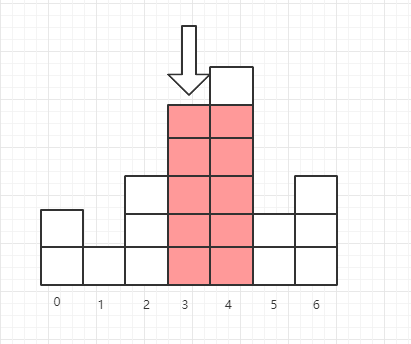从上面的分析可以直到我们可以计算维护一个依次递增的栈，当前发现下一个元素小于目前栈顶最大的元素，我们就开始进行面积计算
Deque<Integer> stack = new ArrayDeque<>();
for (int i = 0; i < heights.length; i++) {
while (!stack.isEmpty() && heights[stack.peek()]>heights[i]) {
int h = heights[stack.peek()];
int left = stack.poll();
int right = i - 1;
ans = Math.max(ans, (right - left + 1) * h);
}
stack.push(i);
}
}


，无法进去循环，不存在 heights[stack.peek()]>heights[i]进行下一个元素的比较
[1,1]，最大面积应该是2，无法进行计算最小元素从左到右的面积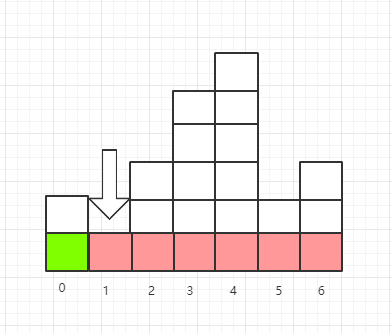int[] newHeights = new int[heights.length + 2];
//这里，原数组，复制的起始位置，新数组，新数组开始的插入的下标，原数组复制的个数
System.arraycopy(heights, 0, newHeights, 1, heights.length);
Deque<Integer> stack = new ArrayDeque<>();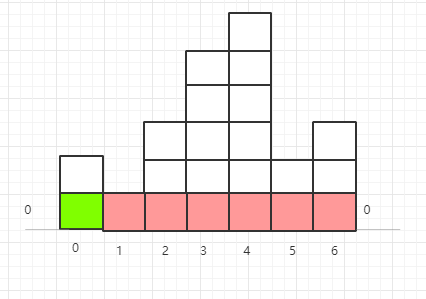for (int i = 0; i < newHeights.length; i++) {
while (!stack.isEmpty() && newHeights[stack.peek()]>newHeights[i]) {
int h = newHeights[stack.pop()];
//有效的数字需要+1
int left = stack.peek() + 1;
int right = i - 1;
ans = Math.max(ans, (right - left + 1) * h);
}


ans = Math.max(ans, ( i - 1- stack.peek()) * h);